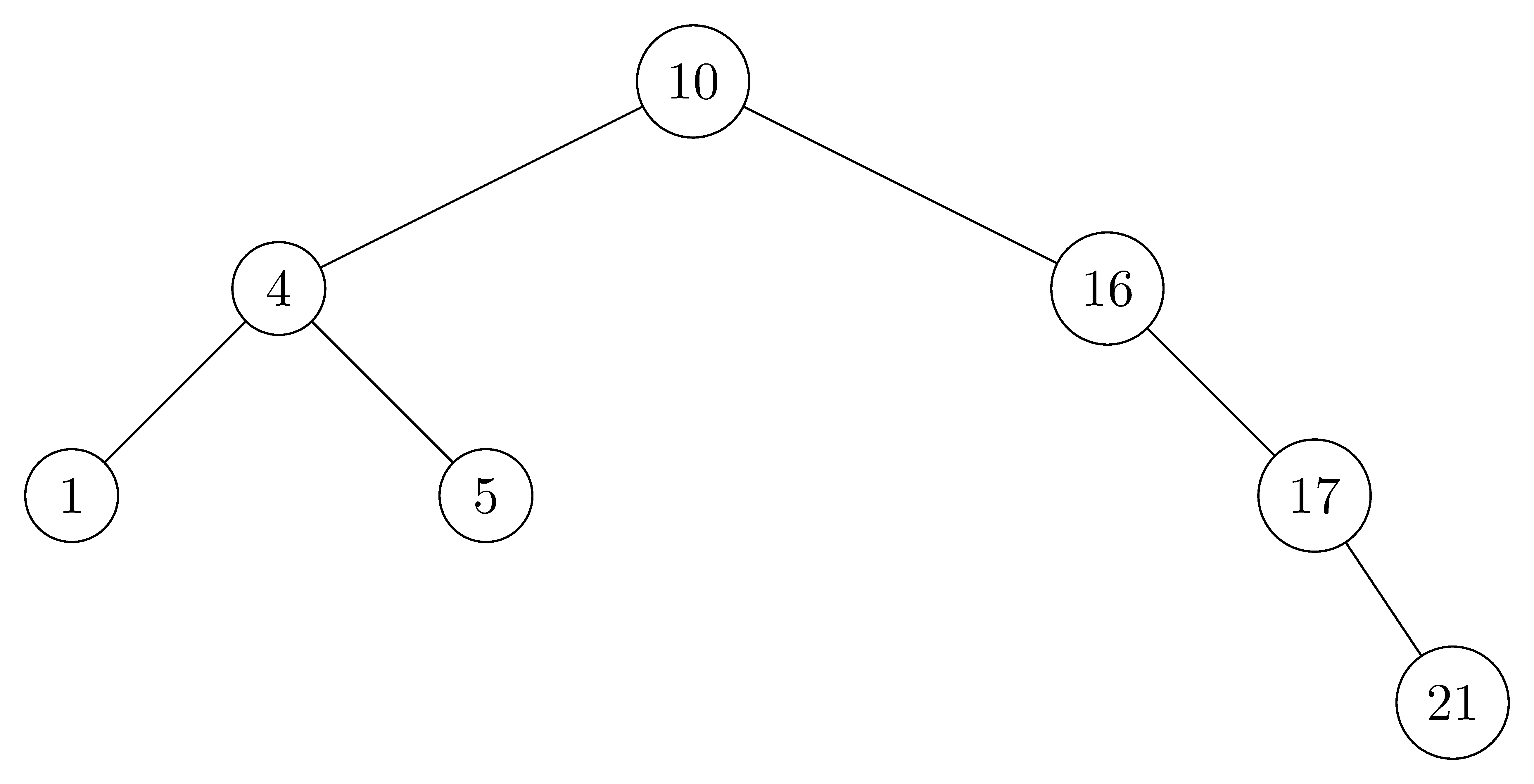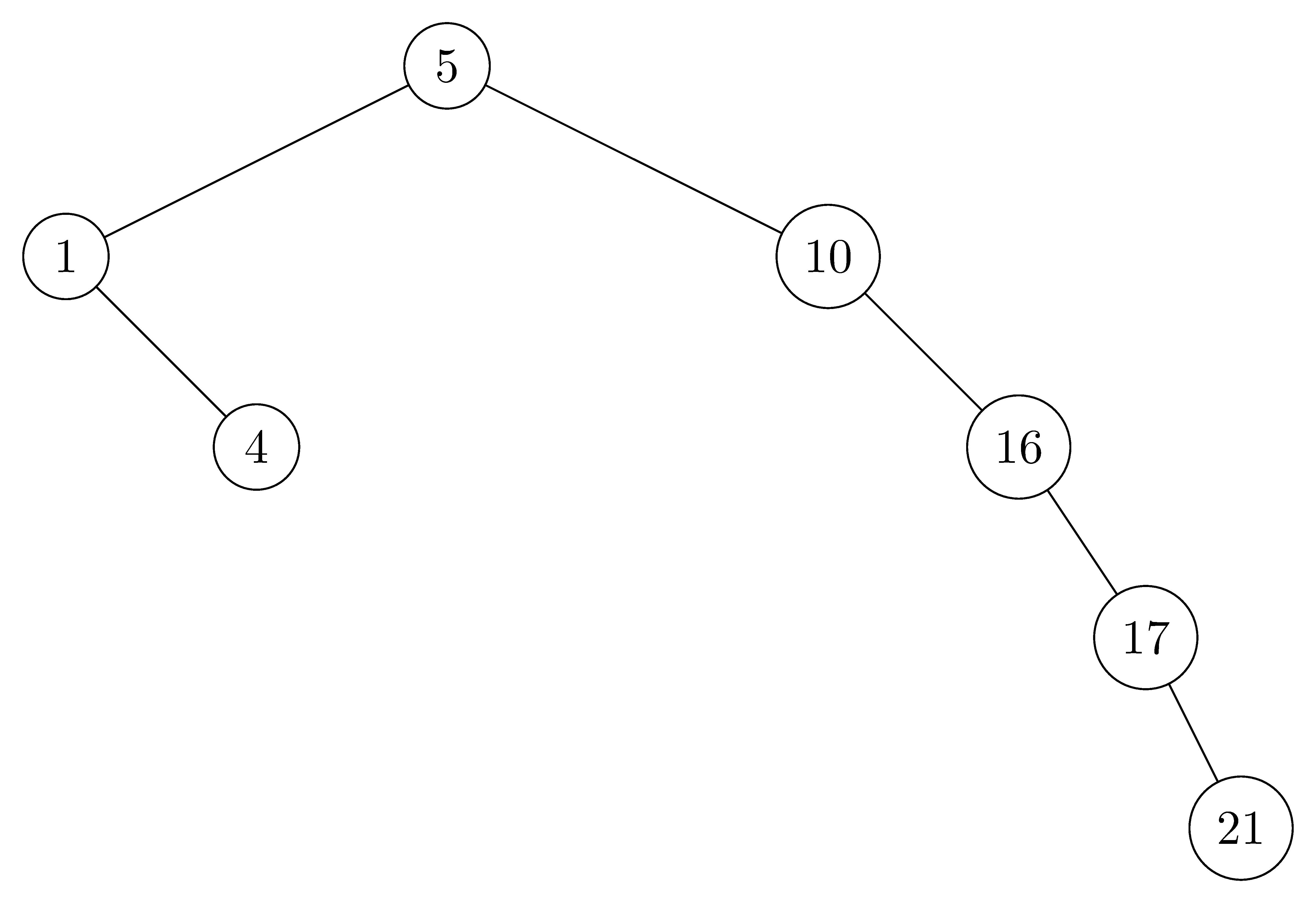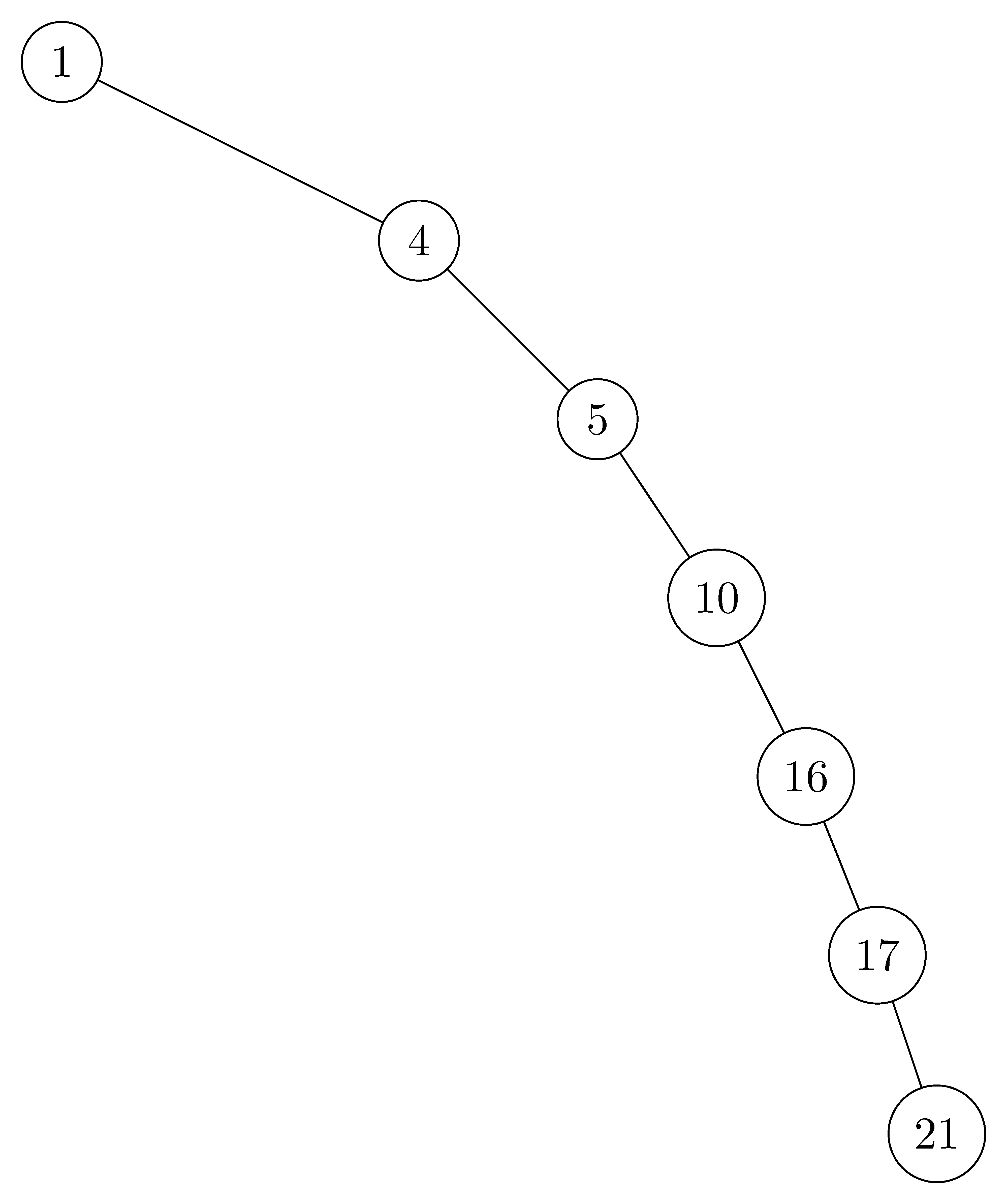# 12.1 What is a binary search tree?

## 12.1-1

For the set of $\{ 1, 4, 5, 10, 16, 17, 21 \}$ of keys, draw binary search trees of heights $2$, $3$, $4$, $5$, and $6$.

• $height = 2$:• $height = 3$:• $height = 4$:• $height = 5$:• $height = 6$:## 12.1-2

What is the difference between the binary-search-tree property and the min-heap property (see page 153)? Can the min-heap property be used to print out the keys of an $n$-node tree in sorted order in $O(n)$ time? Show how, or explain why not.

• The binary-search-tree property guarantees that all nodes in the left subtree are smaller, and all nodes in the right subtree are larger.
• The min-heap property only guarantees the general child-larger-than-parent relation, but doesn't distinguish between left and right children. For this reason, the min-heap property can't be used to print out the keys in sorted order in linear time because we have no way of knowing which subtree contains the next smallest element.

## 12.1-3

Give a nonrecursive algorithm that performs an inorder tree walk. ($\textit{Hint:}$ An easy solution uses a stack as an auxiliary data structure. A more complicated, but elegant, solution uses no stack but assumes that we can test two pointers for equality.)

INORDER-TREE-WALK(T)
let S be an empty stack
current = T.root
done = 0
while !done
if current != NIL
PUSH(S, current)
current = current.left
else
if !S.EMPTY()
current = POP(S)
print current
current = current.right
else done = 1


## 12.1-4

Give recursive algorithms that perform preorder and postorder tree walks in $\Theta(n)$ time on a tree of $n$ nodes.

PREORDER-TREE-WALK(x)
if x != NIL
print x.key
PREORDER-TREE-WALK(x.left)
PREORDER-TREE-WALK(x.right)

POSTORDER-TREE-WALK(x)
if x != NIL
POSTORDER-TREE-WALK(x.left)
POSTORDER-TREE-WALK(x.right)
print x.key


## 12.1-5

Argue that since sorting $n$ elements takes $\Omega(n\lg n)$ time in the worst case in the comparison model, any comparison-based algorithm for constructing a binary search tree from an arbitrary list of $n$ elements takes $\Omega(n\lg n)$ time in the worst case.

Assume, for the sake of contradiction, that we can construct the binary search tree by comparison-based algorithm using less than $\Omega(n\lg n)$ time, since the inorder tree walk is $\Theta(n)$, then we can get the sorted elements in less than $\Omega(n\lg n)$ time, which contradicts the fact that sorting $n$ elements takes $\Omega(n\lg n)$ time in the worst case.